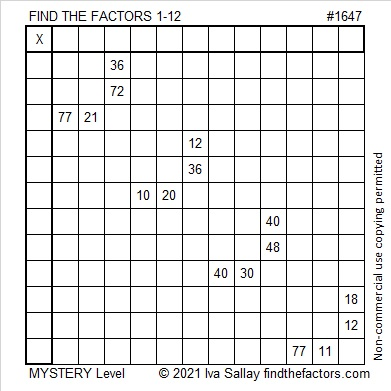# 1647 A Fun Mystery

Contents

### Today’s Puzzle:

I’ve never made a puzzle quite like this one before, and even though the logic needed is a little tricky, I found it quite enjoyable. Share it with a friend and see if you don’t have some great discussions such as if the common factor of 12 and 36 is 3, 4, 6, or 12.### Factors of 1647:

1 + 6 + 4 + 7 = 18, a multiple of 3 and 9, so 1647 is divisible by both 3 and 9.

• 1647 is a composite number.
• Prime factorization: 1647 = 3 × 3 × 3 × 61, which can be written 1647 = 3³ × 61.
• 1647 has at least one exponent greater than 1 in its prime factorization so √1647 can be simplified. Taking the factor pair from the factor pair table below with the largest square number factor, we get √1647 = (√9)(√183) = 3√183.
• The exponents in the prime factorization are 3 and 1. Adding one to each exponent and multiplying we get (3 + 1)(1 + 1) = 4 × 2 = 8. Therefore 1647 has exactly 8 factors.
• The factors of 1647 are outlined with their factor pair partners in the graphic below.### More About the Number 1647:

1647 is the hypotenuse of a Pythagorean triple:
297-1620-1647, which is 27 times (11-60-61).

1647 is a repdigit in base 13:
1647₁₀ = 999₁₃ because
9(13² + 13¹ + 13º) =
9(169 + 13 + 1) =
9(183) = 1647.

This site uses Akismet to reduce spam. Learn how your comment data is processed.